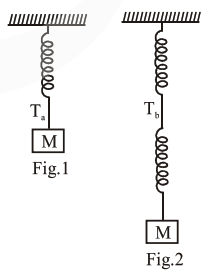# Consider two identicalQuestion:

Consider two identical springs each of spring constant $\mathrm{k}$ and negligible mass compared to the mass $M$ as shown. Fig.1 shows one of them and Fig. 2 shows their series combination. The ratios of time period of oscillation of the two SHM is $\frac{T_{b}}{T_{a}}=\sqrt{x}$, where value of $x$ is

(Round off to the Nearest Integer)Solution:

$\mathrm{T}_{\mathrm{a}}=2 \pi \sqrt{\frac{\mathrm{M}}{\mathrm{K}}}$

$\mathrm{T}_{\mathrm{b}}=2 \pi \sqrt{\frac{\mathrm{M}}{\mathrm{K} / 2}}$

$\frac{\mathrm{T}_{\mathrm{b}}}{\mathrm{T}_{\mathrm{a}}}=\sqrt{2}=\sqrt{\mathrm{x}}$

$\Rightarrow \mathrm{x}=2$